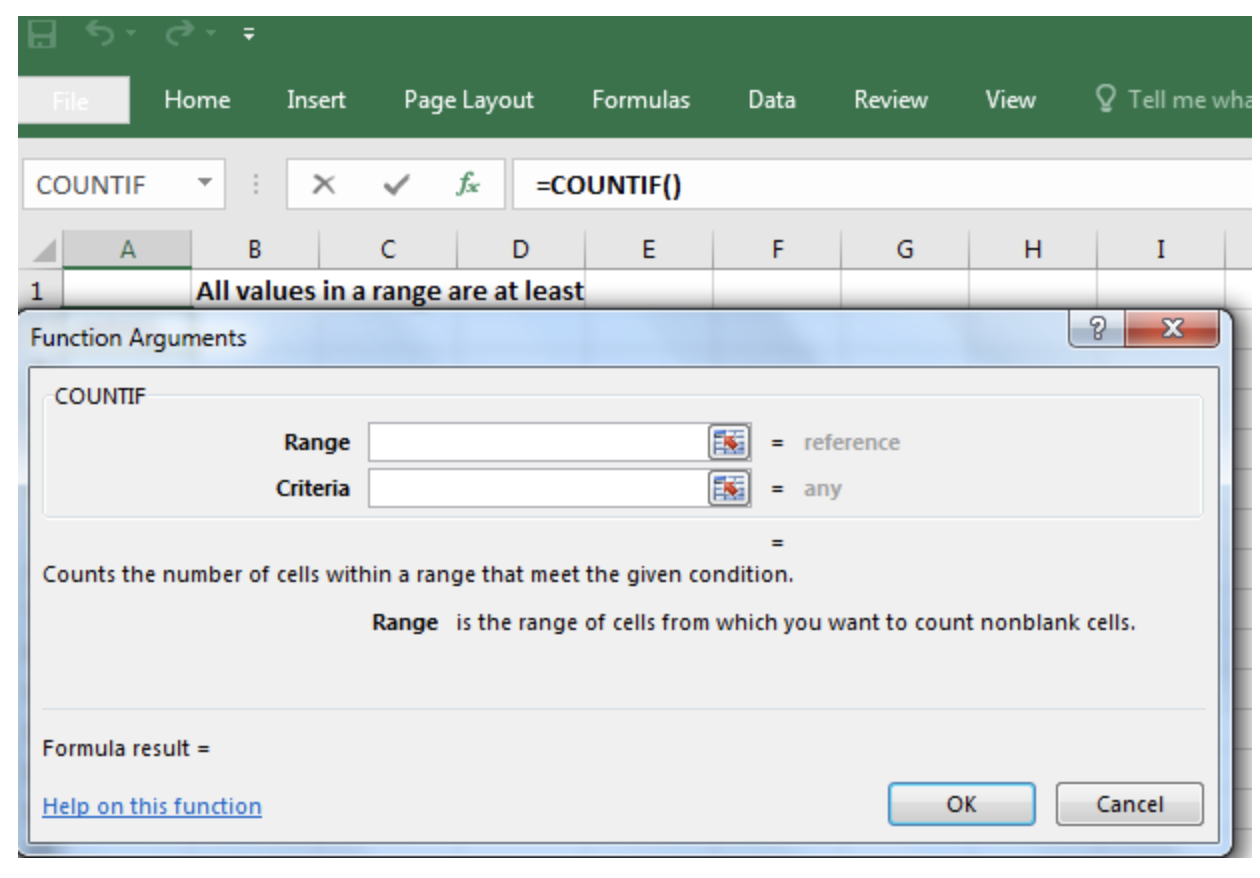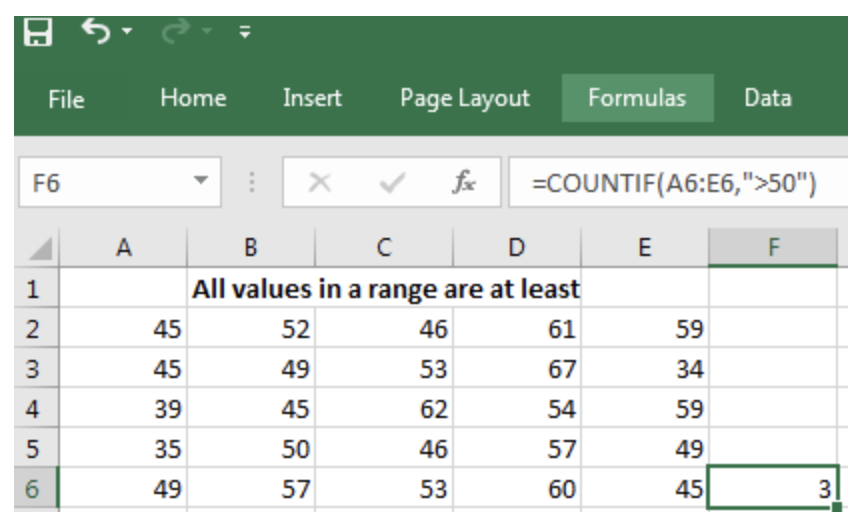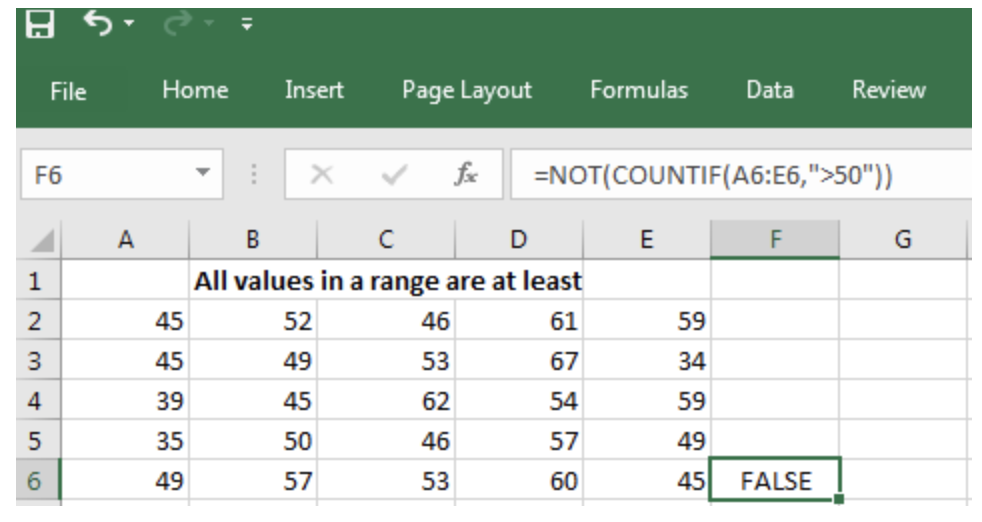Get instant live expert help with Excel or Google Sheets“My Excelchat expert helped me in less than 20 minutes, saving me what would have been 5 hours of work!”

#### Post your problem and you'll get expert help in seconds

Your message must be at least 40 characters
Our professional experts are available now. Your privacy is guaranteed.

# How to Check If All Values in a Range Are at Least a Certain Criteria

Want to learn how to check if values in a range are above a certain threshold? This post will give you an overview of how to do so using the COUNTIF function in Excel.

## How to Check If All Values in a Range Are at Least a Certain Criteria in Excel

### Formula Using COUNTIF

`=COUNTIF(Where do you want to look?, What do you want to look for?)`

To check if values in a range are above a certain threshold using the COUNTIF function, follow the procedure below:

• Click on Formulas on the menu bar in Excel.
• Next, click on More Functions and point the cursor on Statistical.
• Scroll down the list that displays and click on COUNTIF.

### Example of formula

The dialogue box will display as shown below.Figure 1: How to access the COUNTIF formula in Excel

• The range is the span of cells to which you want to apply the formula. In the example below, it is A4 to E4. Type A4:E4.
• The criteria are the value you want to use to determine the entries to be counted. In the example below, the criteria are 50. Type “>50”
• Click on OK.

It will show you the result displayed in the screenshot below:Figure 2: Result displayed by COUNTIF

### Interpretation of the Result

What is displayed above shows that in the row extending from A4 to E4 in the spreadsheet above, using the COUNTIF function to count values that are at least 50, that is, `=COUNTIF``(A4`E4`,“>50”)`, only 3 entries are at least 50. It is easy to look at the table and verify by physically counting that the formula is correct. The only values in that row that are 50 and above are 57, 53 and 60.

Alternatively, simply type the formula above into the cell on the right of the row you are interested in. Hit the Enter key when you finish typing, and the result will show.

NOT can be used in conjunction with the COUNTIF in order to assess whether all the entries in a spreadsheet have at least the chosen minimum value. Consider the example displayed on the screenshot below in which the formula in F4 is: `=NOT(COUNTIF(A4:E4, “>50”))`Figure 3: All the entries in the range have at least the value of 50.

In this case, the formula for F4 indicates that all the entries in the range have at least the value of 50 and above. Since this is not true for the row in question, COUNTIF gives false.

This is the way this formula does it:

What COUNTIF formula does essentially is to count all the cells that have values which are at least up to a certain value, which in the formula above, is fixed as 50. `COUNTIF(A4:E4,">50")`

Here, COUNTIF will give the number of cells that contain an entry that is at least 50. If there is no such cell, it will give zero.

The NOT command is used for displaying the COUNTIF results in True or False format.

• Where any of the entries fall above 50, COUNTIF will state how many there are, while NOT will give False if some of the entries that fall below 50.
• In the same manner, where there are no values that fall above 50, COUNTIF will give zero, while NOT will give True, meaning that all the values are at least 50.

Still need some help with Excel formatting or have other questions about Excel? Connect with a live Excel expert here for some 1 on 1 help. Your first session is always free.

### Did this post not answer your question? Get a solution from connecting with the expert.Another blog reader asked this question today on Excelchat:
Related blogs## Subscribe to Excelchat.coAnother blog reader asked this question today on Excelchat: